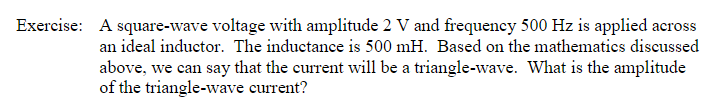# Exercise:A square-wave voltage with amplitude 2 V and frequency 500 Hz is applied acrossan ideal inductor. The inductance is 500 mH. Based on the mathematics discussedabove, we can say that the current will be a triangle-wave. What is the amplitudeof the triangle-wave current?

Question
16 viewshelp_outlineImage TranscriptioncloseExercise: A square-wave voltage with amplitude 2 V and frequency 500 Hz is applied across an ideal inductor. The inductance is 500 mH. Based on the mathematics discussed above, we can say that the current will be a triangle-wave. What is the amplitude of the triangle-wave current? fullscreen
check_circle

Step 1

Amplitude of a square wave is 2 V, frequency is 500 Hz, and inductance is 500 mH

Step 2

The square-wave voltage with amplitude 2 V is shown in Figure 1,

Step 3

### Want to see the full answer?

See Solution

#### Want to see this answer and more?

Solutions are written by subject experts who are available 24/7. Questions are typically answered within 1 hour.*

See Solution
*Response times may vary by subject and question.
Tagged in